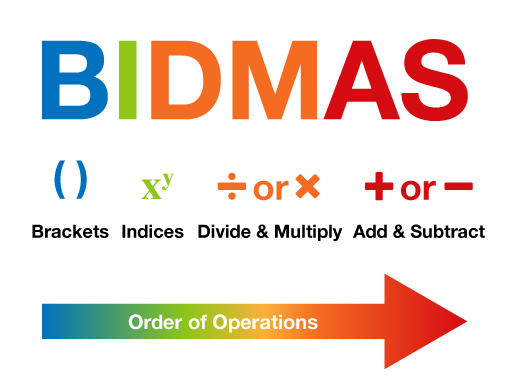Science, Maths & Technology

### Become an OU studentEveryday maths 2

Start this free course now. Just create an account and sign in. Enrol and complete the course for a free statement of participation or digital badge if available.

# 8.1 Order of operations

The order in which you carry out operations can make a big difference to the final answer. When doing any calculation that involves doing more than one operation, you must follow the rules of BIDMAS in order to arrive at the correct answer.Figure 22 The BIDMAS order of operations

## B: Brackets

Any calculation that is in brackets must be done first.

Example:

2 × (3 + 5)

2 × 8 = 16

Note that this could also be written as 2 (3 + 5) because if a number is next to a bracket, it means you need to multiply

## I: Indices

After any calculations in brackets have been done, you must deal with any calculations involving indices or powers i.e.

32 = 3 × 3

or

43 = 4 × 4 × 4

Example:

3 × 42

3 × (4 × 4)

3 × 16 = 48

## D: Divide

Next come any division or multiplication calculations. Of these two calculations, it does not matter which you do first.

Example:

16 − 10 ÷ 5

16 − 2 = 14

## M: Multiply

Example:

5 + 6 × 2

5 + 12 = 17

Finally, any calculations involving addition or subtraction are done. Again, it does not matter which of these two are done first.

Example:

24 + 10 − 2

34 − 2 = 32

or

24 + 8 = 32

## Activity 18: Using BIDMAS

Now have a go at carrying out the following calculations yourself. Make sure you apply BIDMAS!

1. 4 + 3 × 2
2. 5 (4 − 1)
3. 36 ÷ 32
4. 7 + 15 ÷ 3 − 4